41. The minimum number of edges in a connected cyclic graph on n vertices is a. n b. n+1 c. n-1 d. none of the above

 42. The number of edges in a regular graph of degree d and n vertices is a. nd b. n+d c. nd/2 d. maximum of n,d

 43. In the given graph identify the cut vertices.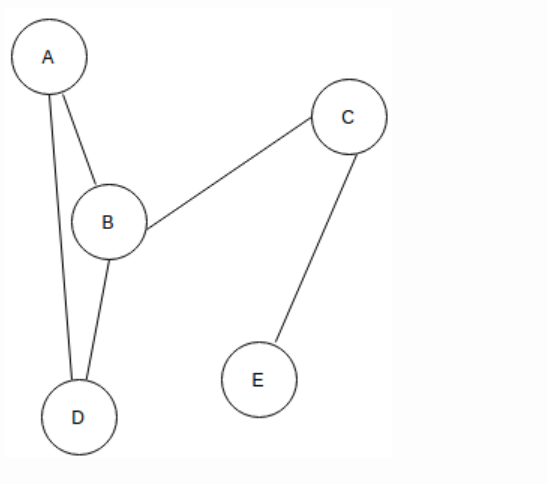a. B and E b. C and D c. A and E d. C and B

 44. For the given graph(G), which of the following statements is true?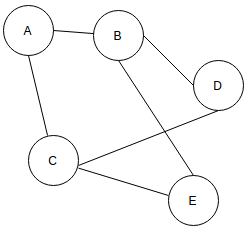a. G is a complete graph b. G is not a connected graph c. The vertex connectivity of the graph is 2 d. The edge connectivity of the graph is 1
 Answer: (c).The vertex connectivity of the graph is 2

 45. The given Graph is regular.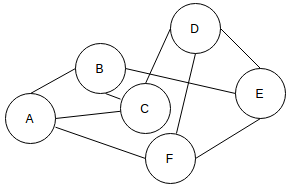a. True b. False c. May be d. Can't say

 46. In the following DAG find out the number of required Stacks in order to represent it in a Graph Structured Stack.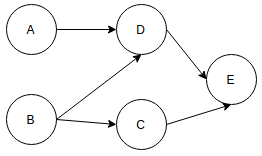a. 1 b. 2 c. 3 d. 4

 47. Which of the following graphs are isomorphic to each other?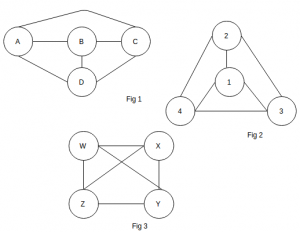a. fig 1 and fig 2 b. fig 2 and fig 3 c. fig 1 and fig 3 d. fig 1, fig 2 and fig 3
 Answer: (d).fig 1, fig 2 and fig 3

 48. In the given graph which edge should be removed to make it a Bipartite Graph?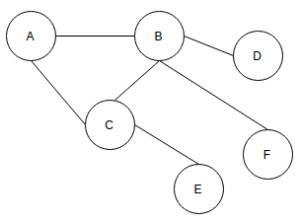a. A-C b. B-E c. C-D d. D-E
 49. What would be the DFS traversal of the given Graph?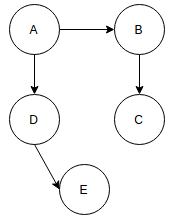a. ABCED b. AEDCB c. EDCBA d. ADECB
 50. What is the number of words that can be formed from the given Directed Acyclic Word Graph?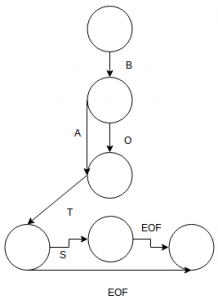a. 2 b. 4 c. 12 d. 7